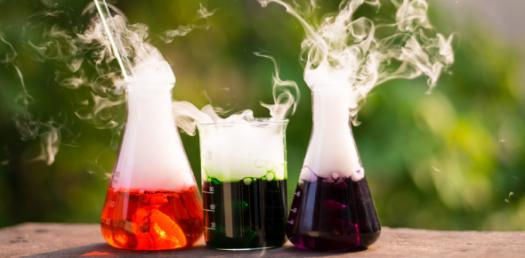# Chemistry Quiz: Can You Pass This Chemical Reaction Test?

10 Questions | Total Attempts: 3250Settings.

• 1.
A true solution is a homogeneous mixture of solute and solvent. Chalk powder in water is a heterogenous mixture. Is it a true solution?
• A.

No. it is not a true solution. It is called Suspension.

• B.

Yes. it is a true solution..

• C.

No. it is not a true solution. It is called Colloid.

• D.

No. it is a true solution.

• 2.
Solution that contains water as the solvent is called aqueous solution. If carbon disulphide is a solvent in a given solution, then the solution is called ______
• A.

Aqueous solution

• B.

Non aqueous solution

• 3.
Solubility of common salt in 100g water is 36g. If 20g of salt is dissolved in it how much more is required to attain saturation?
• A.

36 g of salt

• B.

10 g of salt

• C.

6 g of salt

• D.

16 g of salt

• 4.
If two liquids are mutually soluble, they are called _______ liquids.
• A.

Miscible

• B.

Immiscible

• 5.
When sunlight passes through window of the classrooms its path is visible. This is due to _______of light.
• A.

Reflection

• B.

Scattering

• 6.
The particles in various forms are visible only under ultramicroscope. A solution containing such particles is called __________
• A.

True solution

• B.

Colloidal solution

• 7.
The number of substance present in binary solution is _____
• A.

One

• B.

Two

• 8.
The mixture of gases used by deep sea divers is _______
• A.

Helium-oxygen

• B.

Oxygen-nitrogen

• 9.
Earth soil cannot store more nitrogen than it can hold. Hence earth soil is referred to be in a state of _________.
• A.

Saturation

• B.

Unsaturation

• 10.
In an endothermic process, solubility increases with _________ in temperature.
• A.

Increase

• B.

Decrease

Related TopicsBack to top